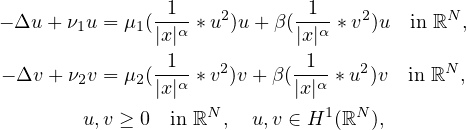Electron. J. Differential Equations, Vol. 2021 (2021), No. 47, pp. 1-20.

### Existence and asymptotic behavior of positive least energy solutions for coupled nonlinear Choquard equations Song You, Peihao Zhao, Qingxuan Wang

Abstract:
In this article, we study the coupled nonlinear Schrödinger equations with Choquard type nonlinearitieswhere ν1212 are positive constants, β>0 is a coupling constant, N≥3, α in (0,N) ∩ (0,4), and "*'" is the convolution operator. We show that the nonlocal elliptic system has a positive least energy solution for positive small β and positive large β via variational methods. For the case in which ν12, μ1≄μ2, N=3,4,5 and α=N-2, we prove the uniqueness of positive least energy solutions. Moreover, the asymptotic behaviors of the positive least energy solutions as β→ 0+ are studied.

Submitted July 17, 2019. Published May 28, 2021.
Math Subject Classifications: 35B40, 35J47, 35J50.
Key Words: Coupled Choquard equations; positive least energy solution; asymptotic behavior; variational method.

Show me the PDF file (399 KB), TEX file for this article.

 Song You Department of Mathematical Sciences Tsinghua University Beijing 100084, China email: yousong@mail.tsinghua.edu.cn Peihao Zhao School of Mathematics and Statistics Lanzhou University Lanzhou, Gansu 730000, China email: zhaoph@lzu.edu.cn Qingxuan Wang Department of Mathematics Zhejiang Normal University Jinhua, Zhejiang 321004, China email: wangqx@zjnu.edu.cn